### Copy to points with random 90 degree rotations

4887   6   1Member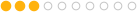63 posts
Joined: Sept. 2014
Offline
I'm using the copy to points node and I want to apply random 90 degree rotations to each copy. My problem is that it appears that the Copy to points node only takes a quaternion rotation matrix as an input. I've played around with the randomize attribute on a float4 ROT using a two value return. From what I can tell a 90 degree rotation in one axis returns a .707 in quaternion coordinates, while a 90 degree rotation in two axis returns a 1 in quaternion coordinates.
Is there a way to use euler rotations in the copy to points node?Member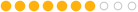201 posts
Joined: July 2015
Offline
These are all the attributes you can use to transform your Instances with the Copy to Points node: LINK [www.sidefx.com]

One convenient way for you would be to create a static up vector and a varying normal vector attribute with different normalized directions.

Matt Estela's cgwiki [www.tokeru.com] should help you out as always.Also look at the Custom Discrete option of the Attribute Randomize SOP [www.sidefx.com] which you can use to create an attribute to split your points into parts. You can then use that attribute to assign different directions to your Normal attribute.
Edited by shadesoforange - Oct. 8, 2020 10:18:43
Manuel Köster - Senior Technical Artist @Remedy Entertainment

https://www.remedygames.com/ [www.remedygames.com]Member63 posts
Joined: Sept. 2014
Offline
Thank you for the awesome response! I'll look through those options.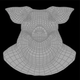Member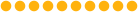1642 posts
Joined: May 2006
Offline

https://www.tokeru.com/cgwiki/index.php?title=JoyOfVex17 [www.tokeru.com]Member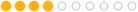77 posts
Joined: Feb. 2017
Offline
oaboraz
Is there a way to use euler rotations in the copy to points node?

yes, you can use eulertoquaternion VEX function to create a quaternion from euler rotation, and then use qmultiply VEX function to multiply this quaternion to orient attribute.

rough code like this:
```vector rotDegree = {90, 0, 0};
int rotOrder = chi("rot_order"); // define a rotate order
vector4 rotQua = eulertoquaternion(radians(rotDegree ), rotOrder);

p@orient = qmultiply(p@orient, rotQua);
```

if you do not want to rotate orient by a quaternion, you also can use maketransform VEX function to create a custom orient / transform directly.

```matrix  maketransform(int trs, int xyz, vector t, vector r, vector s)
```
Edited by zengchen - Oct. 9, 2020 02:40:16Member63 posts
Joined: Sept. 2014
Offline
zengchen
oaboraz
Is there a way to use euler rotations in the copy to points node?

yes, you can use eulertoquaternion VEX function to create a quaternion from euler rotation, and then use qmultiply VEX function to multiply this quaternion to orient attribute.

I'll have to see if that will work for me. I first want to apply a randomize attribute to the points so that when I do a copy to points, each object is facing a random direction, in 90 degree increments.

On a separate note, is there a way to put an attribute directly into a field in a node?
For example in a wrangle if I put
f@move = 5;
f@rotate = 45;
How can I put @move or @rotate into a field on one of the transform nodes? If I just put @move into a field just returns 0.Member77 posts
Joined: Feb. 2017
Offline
oaboraz
On a separate note, is there a way to put an attribute directly into a field in a node?
no, copy to points SOP only can recognise these attributes.
Copying and instancing point attributes [www.sidefx.com]

only orient or transform attribute can set instance's orientation(rotation).

if you want to use @ratate, you must use it to modify orient / transform attribute
for example, apply to orient, rough code like this:
```f@rotate = 45;
vector rotDegree = set(f@rotate, 0, 0);

int rotOrder = chi("rot_order"); // define a rotate order
vector4 rotQua = eulertoquaternion(radians(rotDegree ), rotOrder);

p@orient = qmultiply(p@orient, rotQua);
```
Edited by zengchen - Oct. 9, 2020 12:55:44